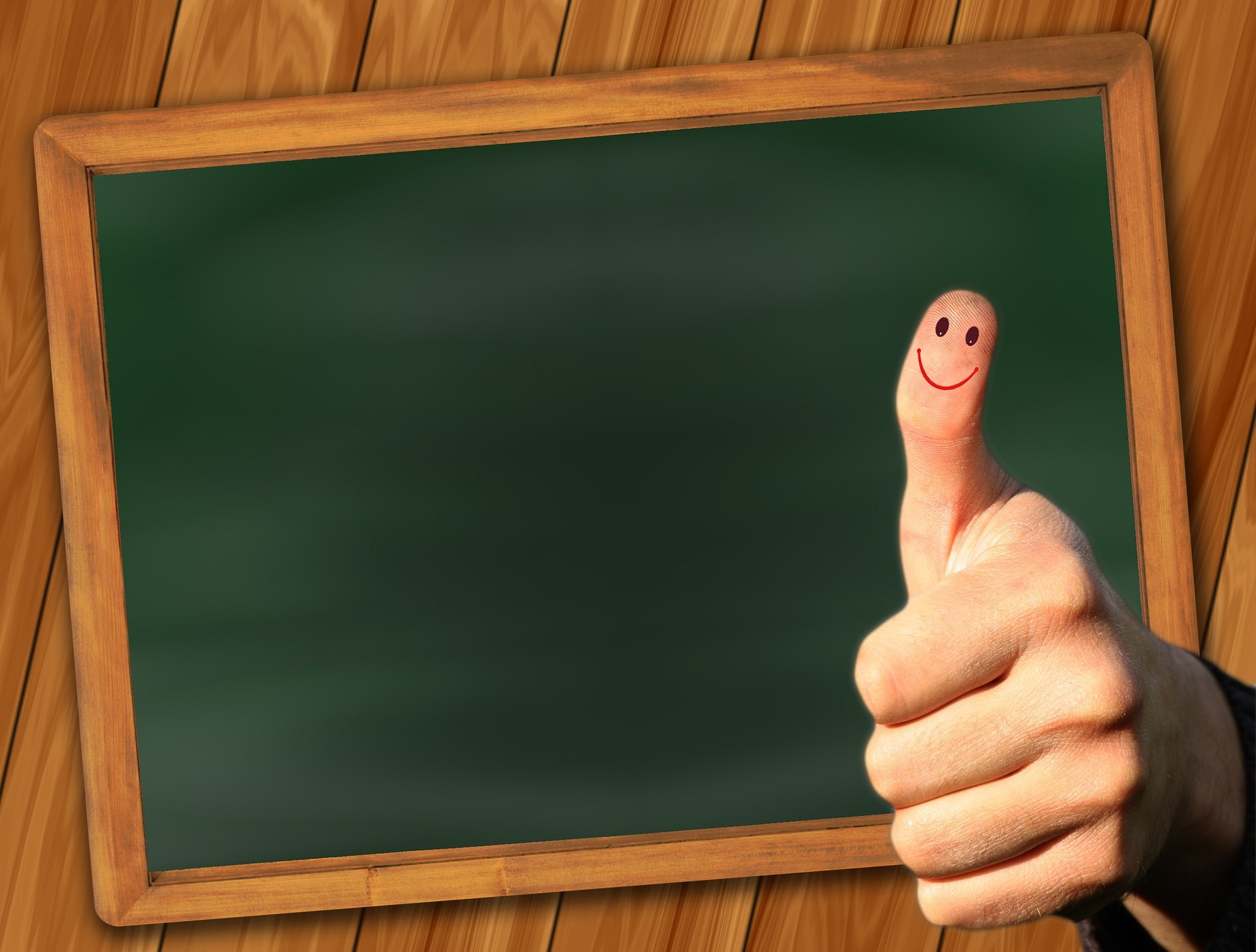# Guiding Your Children Through Primary 1 Maths (Part 3)Now that your children are more comfortable with number relationships, it’s time to move on to more advanced techniques.

1. Adding and subtracting within 10

It’s time to actually add and subtract. Now you can start to have your children plus and minus numbers from each other. Use shapes and toys to do this, and have your children count aloud when they are adding or subtracting. For example, for 3 + 2, have them say something like “three toys plus two toys equals five toys.” Do the same thing with subtraction. They can count using their fingers as well.

1. Introduce multiplication as a concept of adding the same number many times over

Multiplication might be too advanced for your children at this point. However, you can use a more straightforward technique to show them how multiplication works: by adding the same number over and over.

For example, for 3 x 2, put two marbles in three cups each, and have your children slowly add each pair of two marbles together to get the result, meaning 2 + 2 + 2 to get 6. For 5 x 2, put two marbles in five cups each and slowly add each pair of marbles together to get the final result, and so on and so forth.

Once they grasp this concept, memorising multiplication tables will become easier in the long run.

1. Introduce division as a concept of sharing equally

Division is probably an even more advanced concept that your children will have challenge understanding. However, there are ways to make this easier by first showing that division is a way to “share equally.” For example, you can tell them a story: Ah Boon has four sweets to be shared equally with his sister, Ah Gek. How many will he and his sister get? Draw two circles to represent each child, then take four sweets and place them equally in the two circles to show how division is done.

When teaching young children maths, using objects to make an example is more useful, followed by drawing pictures, and once they have a better understanding, you can use maths terms and symbols. Make it more interesting by asking questions that encourage them to find the answer on their own. Remember: maths is about problem-solving, not memory skills or learning procedures. For young children to excel in maths, understanding the relationship of numbers is essential.